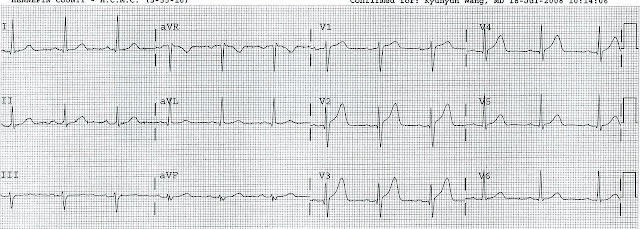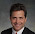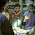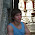## Wednesday, December 31, 2008

### Acute MI from LAD occlusion, or early repolarization?

3 hours of chest pain. Is it STEMI or is it normal (or early repolarization)?

Read Answer Below

This ECG looks quite normal, with only moderate ST elevation, upward concavity, good R-wave amplitude.  There is no ST depression or T-wave inversion.

But one easily identified characteristic makes the ST elevation unlikely to be from early repolarization: the computerized QTc is 455 milliseconds. In my study of early repolarization (ER) (n=167), only 2 of 167 (2%) cases of ER had a QTc greater than 455ms. The mean QTc was 394ms, compared to 420ms for MI (n=125) from LAD occlusion. Conversely, only 4% of LAD occlusion, vs. 40% of ER, had a QTc less than 380 ms. These clues were not appreciated by the clinicians. A very astute interpreter would not that the T-waves are also too symmetric to be early repol, which should have a steeper downslope than upslope, as demonstrated here:

The clinicians did not appreciate these subtle differences.

The patient continued to have chest pain.  70 minutes later, they repeated the ECG, which is shown here:Note that now there are tiny Q-waves in V2-V4, making this unequivocally diagnostic of acute STEMI.
These subtle Q-waves were not appreciated, but the clinicians were astute and ordered a stat echocardiogram, which confirmed anterior wall motion abnormality. The patient was taken to the cath lab and had a 100% LAD occlusion.

After reperfusion, the patients baseline ST-T complex was revealed. This is probably what they would have looked like prior to the LAD occlusion:

For those who want some more detail on differentiating ER from MI, see below:

Also useful was the mean (from V2-V4) R-wave amplitude, at a cutoff of 5 mm, with values less than 5 mm likely to represent MI. Interestingly, mean ST elevation (no matter how it was measured) was not as good a differentiator as the mean R-wave amplitude, but if mean R-wave was less than 5 mm OR the mean ST elevation (V2-V4, as measured at the J-point, STEJ) was greater than or equal to 2 mm, then it was very likely to be MI and very unlikely to be ER.

Even better was a formula derived with logistic regression, which also included the QTc :
(1.196 x STE60 in V3 in mm) + (0.059 x computerized QTc in milliseconds) - (0.326 x RA in V4 in mm), where RA is R-wave amplitude and STE60 is ST elevation at 60ms after the J-point relative to the PR interval.

If the value of the formula is greater than or equal to 23.4, it is MI (Sens, spec, accuracy all around 90%); if less, then it's ER.

For the first ECG, STE60, V3 = 2.5mm, QTc = 455, and RA V4 = 17, so: (1.196 x 2.5) + (0.059 x 455) - ( 0.326 x 17) = 24.29; this is barely greater than 23.4, thus consistent with MI but also further illustrating that this ECG is very difficult.

#### 15 comments:

1.Can you please define the j point - vis the image?

Thanks!

2.The J-point is where the QRS ends and the ST segment begins

1.i wish someone could take a pin and actually point to the "j" point. Seems someone usually points with their finger " its here" . And a figer is so big compared to such a small point, hence, Heisenberg principle follows and difficult for me to find the J point. However, i think it is the point where the s wave ends. And if you ask where this is? well i think this is where the ekg tracing begins to move from vertical to lateral tracing.

2.The J-point is the end of the QRS and beginning of the ST segment. Usually, it is just an inflection point and it is the point where, if you draw a tangent, there is an equal angle between that tangent and the line that comes before and after it. OK?

3.Is it accurate to find another lead (usually lead II or V5 in the rhythm strip beneath the 12 lead) where it is easier to identify the transition from the QRS to the ST segment then draw a vertical line to to identify the exact electrical point in time where the J-point is located on the lead (beat) in question?

3.Thank you, this is very interesting. Using the QT interval is not mentioned as a way of differentiating ischaemia and BER in Rosen's ECG textbook but it does make sense. Do you know the proportion of STEMIs that do not have a prolonged QT?

4.Nice post again!:-)

Did this patient have inferior and lateral wall motion abnormality too? In some cases LAD wraps around the diaphragmatic surface ("long LAD", supplying some part of the inferior wall), and if occluded proximally (before the first diagonal) then the opposite ST vectors of inferior and high lateral injury may cancel each other, and only anterior ST elevation can be seen. So inferior and high-lateral injury both remain silent.

It came to my mind because there is a sign of nonspecific intraventricular conduction disturbance (fragmented QRS) in aVF (which can be a sign of focal block induced my ischaemia, however, can also be seen in normals), and the inferior and high lateral QRS amplitudes diminished compared to the first tracing. Maybe just because of technical things, I don't know.

1.Marton, it was a mid LAD occlusion

5.Dr Smith, i am getting confused about STe at 60ms. Do we calculate ste at j point in lead V3 because if we calculate it at 60 ms it will be same everytime.

1.Sorry, misunderstanding: 60 milliseconds is on the time (x) axis. the voltage, which is what you are measuring, is in millivolts and is on the vertical (y) axis. 1 mm = 0.1 millivolt, but we are making the measurement in millimeters.

So you go to the right 1.5 little boxes (each one is 40 ms), then go up to the tracing line. Then measure how many millimeters it is above an imaginary horizontal line which is drawn over from the PR interval.

2.Yes, that certainly can be helpful.

6.Sir, is there Hyper Acute T in leads V1,V2 and V3 in 1st ECG?

1.Yes, but not everyone recognizes it.

7.I was wondering if the height of T wave matters to differentiate between LAD occlusion and ER. If it does then why the equation doesn't include that as a variable?

1.MG. Read the study if you want to know all about it. The T-waves in both groups were the same height. T-wave height did NOT differentiate. T/R ratio did, because R-waves are small in MI.